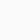# Circuit to turn a servo with 2 inputsTo control a servo motor with three distinct positions (left, middle, right) without using a microcontroller like an Arduino or Raspberry Pi, you can use a dedicated servo controller or a simple electronic circuit with a 555 timer IC.

Here’s an outline of how to achieve this using a 555 timer circuit:

Components Needed:

• Servo motor
• 555 timer IC
• Resistors (for timing)
• Potentiometer (optional, for fine-tuning)
• Capacitors
• Power supply (5-6V)

Circuit Diagram:

plaintext
`+Vcc (5-6V) | R1 | +---|---- OUT to Servo | | | R2 | | +---|---| | | | C1 C2 GND`

Instructions:

1. Connect the positive (+Vcc) and ground (GND) terminals of your power supply to the corresponding points on the circuit.
2. Connect a resistor (R1) between +Vcc and the OUT pin of the 555 timer IC.
3. Connect another resistor (R2) from the OUT pin to the discharge pin (pin 7) of the 555 timer IC.
4. Connect a capacitor (C1) between the discharge pin (pin 7) and ground (GND).
5. Connect another capacitor (C2) between the threshold pin (pin 6) and ground (GND).
6. Connect the servo’s control wire to the OUT pin of the 555 timer IC.
7. Optionally, you can use a potentiometer in place of one of the resistors (R1 or R2) to fine-tune the servo’s positions.
8. Power up the circuit with your chosen voltage source (5-6V is typical for most servo motors).
9. Adjust the values of R1, R2, C1, and C2 to set the timing and pulse width for the three servo positions (left, middle, right). This may require some experimentation.
10. When the circuit is powered on, the 555 timer will generate PWM (Pulse Width Modulation) signals that control the servo’s position.

Remember that the 555 timer circuit will generate a continuous PWM signal, and the servo will hold its position as long as the corresponding PWM pulse is applied. Adjusting the component values will determine the servo’s positions.

This is a basic method to achieve your goal without a microcontroller. However, it might require some trial and error to get the exact positions and timings you want. For precise and more flexible control, using a microcontroller like an Arduino would be a recommended solution.

### PLC-PC communication and control### robot